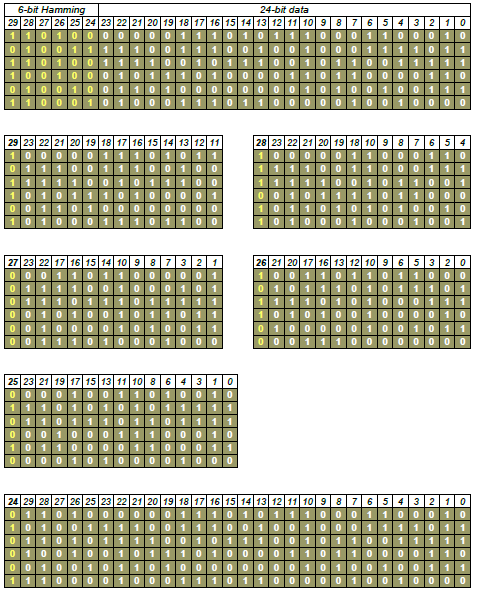## 16 July 2018

The 16 tone frequencies used by CLEW are 605 Hz (used for Doppler correction), 2915 Hz (used for data and synchronization) and 935, 1045, 1155, 1265, 1375, 1485, 1705, 1815, 1925, 2035, 2145, 2255, and 2365 Hz. All information is conveyed by DQPSK modulation at symbol rate of 75 or 45.45 Baud of the 15 data subcarriers tones (Doppler tone remains unmodulated), each of the tone represents 2 data bits and then resulting in a total of 30 data bits consisting of Control Code frames and Data Message frames(M-series messages). The tone at 935 Hz corresponds to bit locations 0 and 1, last tone at 2915 corresponds to bit locations 28 and 29.
Sometimes you may see CLEW using simultaneously the USB and LSB (ISB): indeed, identical signals are transmitted on in ISB and at the receiver both the sidebands will be separately and independently deodulated.  A means is provided to allow operator selection of USB, LSB or the diversity operation (DIV) modes:
• DIV: data words derived from the diversity combination of the USB and LSB will be provided to the tactical computer. This mode of operation is used to help combat multipath interference.
• Automatic: the receiving station automatically selects the version that represents the best information available from the USB, LSB or DIV version of the received data word.
When the criteria do not establish a clear choice, the DIV version is selected.

The three control codes which are used to operate a Link-11 net are the Start Code, Stop Code, and Address Code, each consisting of two encoded 30-bit frames. The Start Code is the first two frames of the transmitted data message while the two frames Stop Code immediately follows the last message frame to signify the end of the data. There are two stop codes according to the transmitting station: Control Stop Code (transmission from the data net control station, or DNCS) is a 2 frames consisting of all "zeros", and Picket Stop Code (transmission from a picket station) which consists of a 2 frames consisting of all "ones".
The Start Code is the first two frames of the transmitted data message while the two frames Stop Code immediately follows the last message frame to signify the end of the data. There are two unique stop codes according to the transmitting station:
• Control Stop Code (transmission from the data net control station, or DNCS) is a 2 frames consisting of all "zeros",
• Picket Stop Code (transmission from a picket station) is a 2 frames consisting of all "ones".
Each net partecipating unit is identified by the Address Code which immediately follows the Control Stop Code if the DNCS transmits data.Fig. 1 - Link-11 data segment
The Data Message frames (Figure 2) follow the Start Code and contain tactical information. Each Data Message frame consists of 30 bits composed of a 24-bit word provided by the tactical computer and 6 Hamming parity bits (bit locations 24 through 29) provided by the modem. The Hamming parity bits are used by the receiver modem for error detection and correction, for this reason they are also refferred to as EDAC bits. Only Data Messages contain Hamming parity bits: Start, Stop, and Address codes are not Hamming parity encoded.
Notice that the 24-bit words may be optionally encrypted using KG-40 crypto device which just sits in midlle between tactical computer and modem.Fig. 2 - Link-11 Data Message frames
The six EDAC bits are encoded as follows:
• Bit 29 is set such that when added to bits locations in 11 through 23, the number of ones will be an odd number;
• Bit 28 is set such that when added to bits locations in 4 through 10 and 18 through 23, the number of ones will be an odd number;
• Bit 27 is set such that when added to bits in locations 1,2,3,7,8,9,10,14,15,16,17,22, and 23, the number of ones will be an odd number;
• Bit 26 is set such that when added to bits in locations 0,2,3,5,6,9,10,12,13,16,17,20, and 21, the number of ones will be an odd number;
• Bit 25 is set such that when added to bits in locations 0,1,3,4,6,8,10,11,13,15,17,19,21, and 23,the number of ones will be an odd number;
• Bit 24 is set such that when all the bits of the frame are added, the number of ones will be an odd number. Bit 24 is called the overall parity bit.
Figure 3 shows a check of the first six 30-bit frames of the Data Message in Fig.2Fig. 3

For who loves GNU Octave,  my friend Christoph wrote a macro just to check Hamming parity in Link-11 Data Message frames:

## -*- octave -*-
fid = fopen(fn);
s=[];
try
i=1;
while true
s(i++,:)=fgetl(fid);
end
end
fclose(fid);

a = s=='1';
## reverse order
a = a(:, end:-1:1);

## constraints
k{1} = [1:30];
k{2} = [1 2 4 5 7 9 11 12 14 16 18 20 22 24 26];
k{3} = [1 3 4 6 7 10 11 13 14 17 18 21 22 27];
k{4} = [2:4 8:11 15:18 23 24 28];
k{5} = [5:11 19:24 29];
k{6} = [12:24 30];

for i=1:6
x(i,1) = sum(mod(sum(a(:,k{i})'),2) == 1);
end
x /= size(a,1);

printf('success: %d %.2f%%\n', [[1:6]' 100*x]');
endfunction